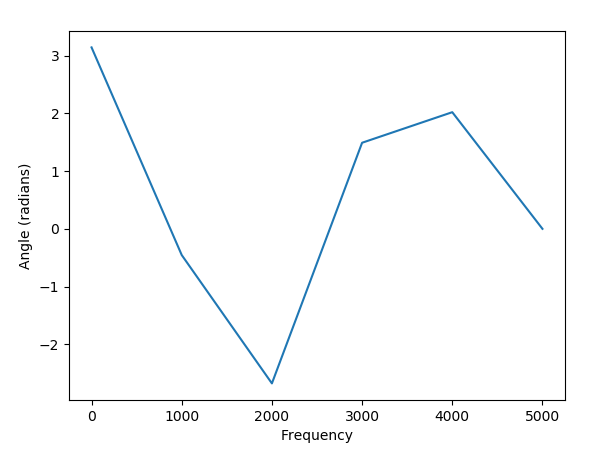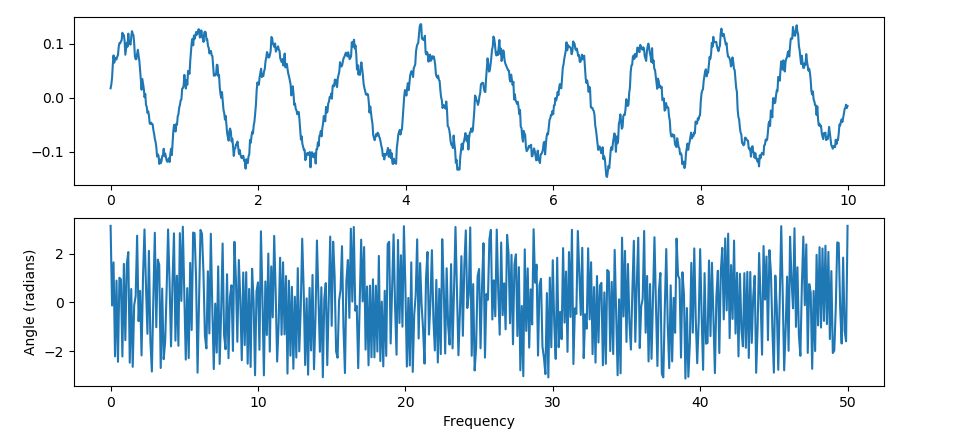# matplotlib.pyplot.angle_spectrum() in Python

Matplotlib is a library in Python and it is numerical – mathematical extension for NumPy library. Pyplot is a state-based interface to a Matplotlib module which provides a MATLAB-like interface.

## matplotlib.pyplot.acorr() Function:

The angle_spectrum() function in pyplot module of matplotlib library is used to plot the angle spectrum. Generally, it computes the angle spectrum of sequence and plotting is done.

Syntax: angle_spectrum(x, Fs=2, Fc=0, window=mlab.window_hanning, pad_to=None, sides=’default’, **kwargs)

Parameters: This method accept the following parameters that are described below:

• x: This parameter is a sequence of data.
• Fs : This parameter is a scalar. Its default value is 2.
• window: This parameter take a data segment as an argument and return the windowed version of the segment. Its default value is window_hanning()
• sides: This parameter specifies which sides of the spectrum to return. This can have following values : ‘default’, ‘onesided’ and ‘twosided’.
• pad_to : This parameter contains the integer value to which the data segment is padded.
• Fc: This parameter is also contains the integer value to offsets the x extents of the plot to reflect the frequency range. Its default value is 0

Returns: This returns the following:

• spectrum :This returns the angle spectrum in radians.
• freqs :This returns the frequencies corresponding to the elements in spectrum.
• line : This returns the line created by this function.

The resultant is (spectrum, freqs, line)

Below examples illustrate the matplotlib.pyplot.angle_spectrum() function in matplotlib.pyplot:

Example #1:

 `# Implementation of matplotlib.pyplot.angle_spectrum() ` `# function ` ` `  `import` `matplotlib.pyplot as plt ` `import` `numpy as np ` ` `  `np.random.seed(``10``*``*``5``) ` ` `  `dt ``=` `0.0001` `Fs ``=` `1` `/` `dt ` `geeks ``=` `np.array([``24.40``, ``110.25``, ``20.05``,  ` `                  ``22.00``, ``61.90``, ``7.80``,  ` `                  ``15.00``, ``22.80``, ``34.90``,  ` `                  ``57.30``]) ` ` `  `nse ``=` `np.random.randn(``len``(geeks)) ` `r ``=` `np.exp(``-``geeks ``/` `0.05``) ` ` `  `s ``=` `0.1` `*` `np.sin(``2` `*` `np.pi ``*` `geeks) ``+` `nse ` ` `  `# plot angle_spectrum ` `plt.angle_spectrum(s, Fs ``=` `Fs) ` ` `  `# Display the simple spectrum and  ` `# angle_spectrum plot ` `plt.show() `

Output:Example #2:

 `# Implementation of matplotlib.pyplot.angle_spectrum() ` `# function ` ` `  `import` `matplotlib.pyplot as plt ` `import` `numpy as np ` ` `  `np.random.seed(``0``) ` ` `  `dt ``=` `0.01` `Fs ``=` `1` `/` `dt ` `t ``=` `np.arange(``0``, ``10``, dt) ` `nse ``=` `np.random.randn(``len``(t)) ` `r ``=` `np.exp(``-``t ``/` `0.05``) ` ` `  `cnse ``=` `np.convolve(nse, r)``*``dt ` `cnse ``=` `cnse[:``len``(t)] ` `s ``=` `0.1` `*` `np.sin(``2` `*` `np.pi ``*` `t) ``+` `cnse ` ` `  `# plot simple spectrum ` `plt.subplot(``2``, ``1``, ``1``) ` `plt.plot(t, s) ` ` `  `# plot angle_spectrum ` `plt.subplot(``2``, ``1``, ``2``) ` `plt.angle_spectrum(s, Fs ``=` `Fs) ` ` `  `# Display the simple spectrum and  ` `# angle_spectrum plot ` `plt.show() `

Output:Attention geek! Strengthen your foundations with the Python Programming Foundation Course and learn the basics.

To begin with, your interview preparations Enhance your Data Structures concepts with the Python DS Course.

My Personal Notes arrow_drop_upSmall things always make you to think big

If you like GeeksforGeeks and would like to contribute, you can also write an article using contribute.geeksforgeeks.org or mail your article to contribute@geeksforgeeks.org. See your article appearing on the GeeksforGeeks main page and help other Geeks.

Please Improve this article if you find anything incorrect by clicking on the "Improve Article" button below.

Article Tags :

Be the First to upvote.

Please write to us at contribute@geeksforgeeks.org to report any issue with the above content.